# Package Vignette

This vignette covers the entire adversarial random forest (ARF) pipeline, from model training to parameter learning, density estimation, and data synthesis.

The ARF algorithm is an iterative procedure. In the first instance, we generate synthetic data by independently sampling from the marginals of each feature and training a random forest (RF) to distinguish original from synthetic samples. If accuracy is greater than $$0.5 + \delta$$ (where delta is a user-controlled tolerance parameter, generally set to 0), we create a new dataset by sampling from the marginals within each leaf and training another RF classifier. The procedure repeats until original and synthetic samples cannot be reliably distinguished. With the default verbose = TRUE, the algorithm will print accuracy at each iteration.

# Load libraries
library(arf)
library(data.table)
library(ggplot2)

# Set seed
set.seed(123, "L'Ecuyer-CMRG")

# Train ARF
#> Iteration: 0, Accuracy: 86.53%
#> Iteration: 1, Accuracy: 44.44%
#> Warning: executing %dopar% sequentially: no parallel backend registered

The printouts can be turned off by setting verbose = FALSE. Accuracy is still stored within the arf object, so you can evaluate convergence after the fact. The warning appears just once per session. It can be suppressed by setting parallel = FALSE or registering a parallel backend (more on this below).

# Train ARF with no printouts
arf <- adversarial_rf(iris, verbose = FALSE)

# Plot accuracy against iterations (model converges when accuracy <= 0.5)
tmp <- data.frame('acc' = arf$acc, 'iter' = seq_len(length(arf$acc)))
ggplot(tmp, aes(iter, acc)) +
geom_point() +
geom_path() +
geom_hline(yintercept = 0.5, linetype = 'dashed', color = 'red')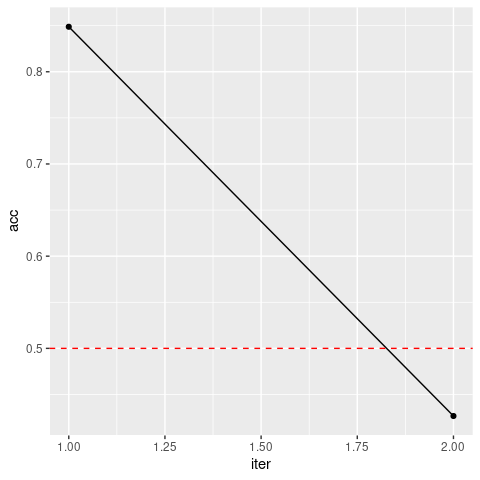We find a quick drop in accuracy following the resampling procedure, as desired. If the ARF has converged, then resulting splits should form fully factorized leaves, i.e. subregions of the feature space where variables are locally independent.

ARF convergence is almost surely guaranteed as $$n \rightarrow \infty$$ (see Watson et al., 2022, Thm. 1). However, this has no implications for finite sample performance. In practice, we often find that adversarial training completes in just one or two rounds, but this may not hold for some datasets. To avoid infinite loops, users can increase the slack parameter delta or set the max_iters argument (default = 10). In addition to these failsafes, adversarial_rf uses early stopping by default (early_stop = TRUE), which terminates training if factorization does not improve from one round to the next. This is recommended, since discriminator accuracy rarely falls much lower once it has increased.

For density estimation tasks, we recommend increasing the default number of trees. We generally use 100 in our experiments, though this may be suboptimal for some datasets. Likelihood estimates are not very sensitive to this parameter above a certain threshold, but larger models incur extra costs in time and memory. We can speed up computations by registering a parallel backend, in which case ARF training is distributed across cores using the ranger package. Much like with ranger, the default behavior of adversarial_rf is to compute in parallel if possible. How exactly this is done varies across operating systems. The following code works on Unix machines.

# Register cores - Unix
library(doParallel)
registerDoParallel(cores = 2)

Windows requires a different setup.

# Register cores - Windows
library(doParallel)
cl <- makeCluster(2)
registerDoParallel(cl)

In either case, we can now execute in parallel.

# Rerun ARF, now in parallel and with more trees
arf <- adversarial_rf(iris, num_trees = 100)
#> Iteration: 0, Accuracy: 92.33%
#> Iteration: 1, Accuracy: 28%

The result is an object of class ranger, which we can input to downstream functions.

# Parameter Learning

The next step is to learn the leaf and distribution parameters using forests for density estimation (FORDE). This function calculates the coverage, bounds, and pdf/pmf parameters for every variable in every leaf. This can be an expensive computation for large datasets, as it requires $$\mathcal{O}\big(B \cdot d \cdot n \cdot \log(n)\big)$$ operations, where $$B$$ is the number of trees, $$d$$ is the data dimensionality, and $$n$$ is the sample size. Once again, the process is parallelized by default.

# Compute leaf and distribution parameters
params <- forde(arf, iris)

Default behavior is to use a truncated normal distribution for continuous data (with boundaries given by the tree’s split parameters) and a multinomial distribution for categorical data. We find that this produces stable results in a wide range of settings. You can also use a uniform distribution for continuous features by setting family = 'unif', thereby instantiating a piecewise constant density estimator.

# Recompute with uniform density
params_unif <- forde(arf, iris, family = 'unif')

This method tends to perform poorly in practice, and we do not recommend it. The option is implemented primarily for benchmarking purposes. Alternative families, e.g. truncated Poisson or beta distributions, may be useful for certain problems. Future releases will expand the range of options for the family argument.

The alpha and epsilon arguments allow for optional regularization of multinomial and uniform distributions, respectively. These help prevent zero likelihood samples when test data fall outside the support of training data. The former is a pseudocount parameter that applies Laplace smoothing within leaves, preventing unobserved values from being assigned zero probability unless splits explicitly rule them out. In other words, we impose a flat Dirichlet prior $$\text{Dir}(\alpha)$$ and report posterior probabilities rather than maximum likelihood estimates. The latter is a slack parameter on empirical bounds that expands the estimated extrema for continuous features by a factor of $$1 + \epsilon$$.

Compare the results of our original probability estimates for the Species variable with those obtained by adding a pseudocount of $$\alpha = 0.1$$.

# Recompute with additive smoothing
params_alpha <- forde(arf, iris, alpha = 0.1)

# Compare results
head(params$cat) #> f_idx variable val prob #> 1: 1 Species virginica 1.0000000 #> 2: 2 Species setosa 1.0000000 #> 3: 3 Species versicolor 0.6000000 #> 4: 3 Species virginica 0.4000000 #> 5: 4 Species setosa 1.0000000 #> 6: 5 Species versicolor 0.3333333 head(params_alpha$cat)
#>    f_idx variable        val        prob
#> 1:     1  Species versicolor 0.008849558
#> 2:     1  Species  virginica 0.982300885
#> 3:     1  Species     setosa 0.008849558
#> 4:     2  Species versicolor 0.008849558
#> 5:     2  Species  virginica 0.008849558
#> 6:     2  Species     setosa 0.982300885

Under Laplace smoothing, extreme probabilities only occur when the splits explicitly demand it. Otherwise, all values shrink toward a uniform prior. Note that these two data tables may not have exactly the same rows, as we omit zero probability events to conserve memory. However, we can verify that probabilities sum to unity for each leaf-variable combination.

# Sum probabilities
summary(params$cat[, sum(prob), by = .(f_idx, variable)]$V1)
#>    Min. 1st Qu.  Median    Mean 3rd Qu.    Max.
#>       1       1       1       1       1       1
summary(params_alpha$cat[, sum(prob), by = .(f_idx, variable)]$V1)
#>    Min. 1st Qu.  Median    Mean 3rd Qu.    Max.
#>       1       1       1       1       1       1

The forde function outputs a list of length 5, with entries for (1) continuous features; (2) categorical features; (3) leaf parameters; (4) variable metadata; and (5) data input class.

params
#> $cnt #> f_idx variable min max mu sigma #> 1: 1 Petal.Length -Inf Inf 6.336364 0.35006493 #> 2: 1 Petal.Width -Inf Inf 2.045455 0.26594600 #> 3: 1 Sepal.Length 7.15 Inf 7.509091 0.25477263 #> 4: 1 Sepal.Width -Inf Inf 3.136364 0.41538591 #> 5: 2 Petal.Length -Inf 1.35 1.236364 0.10269106 #> --- #> 7396: 1849 Sepal.Width 2.95 Inf 3.000000 0.01869639 #> 7397: 1850 Petal.Length 5.05 Inf 5.366667 0.23094011 #> 7398: 1850 Petal.Width -Inf 1.90 1.800000 0.70201186 #> 7399: 1850 Sepal.Length -Inf 6.75 6.266667 0.32145503 #> 7400: 1850 Sepal.Width 2.95 Inf 3.033333 0.05773503 #> #>$cat
#>       f_idx variable        val prob
#>    1:     1  Species  virginica  1.0
#>    2:     2  Species     setosa  1.0
#>    3:     3  Species versicolor  0.6
#>    4:     3  Species  virginica  0.4
#>    5:     4  Species     setosa  1.0
#>   ---
#> 2341:  1846  Species  virginica  1.0
#> 2342:  1847  Species versicolor  1.0
#> 2343:  1848  Species versicolor  1.0
#> 2344:  1849  Species  virginica  1.0
#> 2345:  1850  Species  virginica  1.0
#>
#> $forest #> f_idx tree leaf cvg #> 1: 1 1 17 0.07333333 #> 2: 2 1 18 0.07333333 #> 3: 3 1 27 0.03333333 #> 4: 4 1 30 0.06000000 #> 5: 5 1 33 0.02000000 #> --- #> 1846: 1846 100 67 0.07333333 #> 1847: 1847 100 71 0.05333333 #> 1848: 1848 100 74 0.03333333 #> 1849: 1849 100 76 0.01333333 #> 1850: 1850 100 77 0.02000000 #> #>$meta
#>        variable   class    family
#> 1: Sepal.Length numeric truncnorm
#> 2:  Sepal.Width numeric truncnorm
#> 3: Petal.Length numeric truncnorm
#> 4:  Petal.Width numeric truncnorm
#> 5:      Species  factor  multinom
#>
#> $input_class #>  "data.frame" These parameters can be used for a variety of downstream tasks, such as likelihood estimation and data synthesis. # Likelihood Estimation To calculate log-likelihoods, we pass arf and params on to the lik function, along with the data whose likelihood we wish to evaluate. # Compute likelihood under truncated normal and uniform distributions ll <- lik(arf, params, iris) ll_unif <- lik(arf, params_unif, iris) # Compare average negative log-likelihood (lower is better) -mean(ll) #>  0.3133987 -mean(ll_unif) #>  3.805665 Note that the piecewise constant estimator does considerably worse in this experiment. We can compute likelihoods on the probability scale by setting log = FALSE, but this may result in numerical underflow. There is also a batch argument, which has no impact on results but can be more memory efficient for large datasets. For instance, we could rerun the code above in batches of size 50: # Compute likelihood in batches of 50 ll_50 <- lik(arf, params, iris, batch = 50) # Identical results? identical(ll, ll_50) #>  TRUE In this example, we have used the same data throughout. This may lead to overfitting. With sufficient data, it is preferable to use a training set for adversarial_rf, a validation set for forde, and a test set for lik. Alternatively, we can set the oob argument to TRUE for either of the latter two functions, in which case computations are performed only on out-of-bag (OOB) data. These are samples that are randomly excluded from a given tree due to the bootstrapping subroutine of the RF classifier. Note that this only works when the dataset x passed to forde or lik is the same one used to train the arf. Recall that a sample’s probability of being excluded from a single tree is $$e^{-1} \approx 0.368$$. When using oob = TRUE, be sure to include enough trees so that every observation is likely to be OOB at least a few times. # Data synthesis For this experiment, we use the smiley simulation from the mlbench package, which allows for easy visual assessment. We draw a training set of $$n = 1000$$ and simulate $$1000$$ synthetic datapoints. Resulting data are plotted side by side. # Simulate training data library(mlbench) x <- mlbench.smiley(1000) x <- data.frame(x$x, x$classes) colnames(x) <- c('X', 'Y', 'Class') # Fit ARF arf <- adversarial_rf(x, mtry = 2) #> Iteration: 0, Accuracy: 90.74% #> Iteration: 1, Accuracy: 37.73% # Estimate parameters params <- forde(arf, x) # Simulate data synth <- forge(params, n_synth = 1000) # Compare structure str(x) #> 'data.frame': 1000 obs. of 3 variables: #>$ X    : num  -0.87 -0.7 -0.827 -0.905 -0.751 ...
#>  $Y : num 1.032 0.778 1.068 1.137 1.083 ... #>$ Class: Factor w/ 4 levels "1","2","3","4": 1 1 1 1 1 1 1 1 1 1 ...
str(synth)
#> 'data.frame':    1000 obs. of  3 variables:
#>  $X : num -0.878648 -0.847801 -0.488739 0.76994 0.000893 ... #>$ Y    : num  0.862 1.052 -0.786 0.871 0.693 ...
#>  $Class: Factor w/ 4 levels "1","2","3","4": 1 1 4 2 3 3 3 3 2 4 ... # Put it all together x$Data <- 'Original'
synth$Data <- 'Synthetic' df <- rbind(x, synth) # Plot results ggplot(df, aes(X, Y, color = Class, shape = Class)) + geom_point() + facet_wrap(~ Data)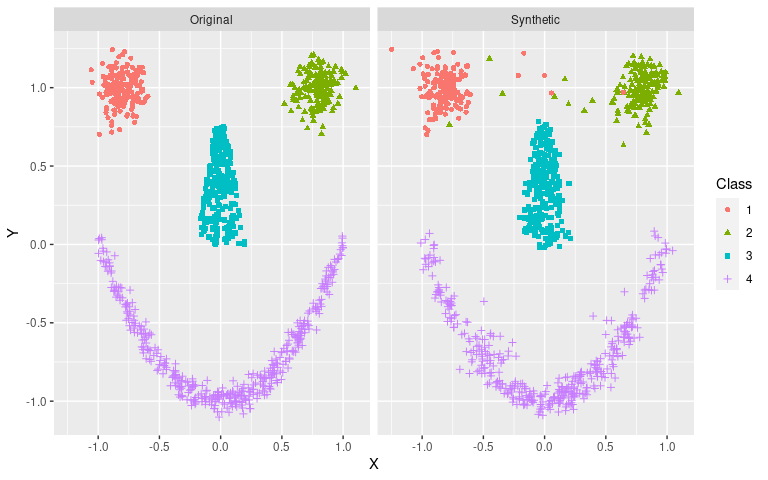The general shape is clearly recognizable, even if some stray samples are evident and borders are not always crisp. This can be improved with more training data. Note that the default behavior of adversarial_rf is to treat integers as ordered factors, with a warning. This makes sense for, say, count data with limited support (e.g., number of petals on a flower). However, this is probably not the desired behavior for other integer variables. Consider the diamonds dataset, where price is classed as an integer. # Check data head(diamonds) #> # A tibble: 6 × 10 #> carat cut color clarity depth table price x y z #> <dbl> <ord> <ord> <ord> <dbl> <dbl> <int> <dbl> <dbl> <dbl> #> 1 0.23 Ideal E SI2 61.5 55 326 3.95 3.98 2.43 #> 2 0.21 Premium E SI1 59.8 61 326 3.89 3.84 2.31 #> 3 0.23 Good E VS1 56.9 65 327 4.05 4.07 2.31 #> 4 0.29 Premium I VS2 62.4 58 334 4.2 4.23 2.63 #> 5 0.31 Good J SI2 63.3 58 335 4.34 4.35 2.75 #> 6 0.24 Very Good J VVS2 62.8 57 336 3.94 3.96 2.48 # View the distribution hist(diamonds$price)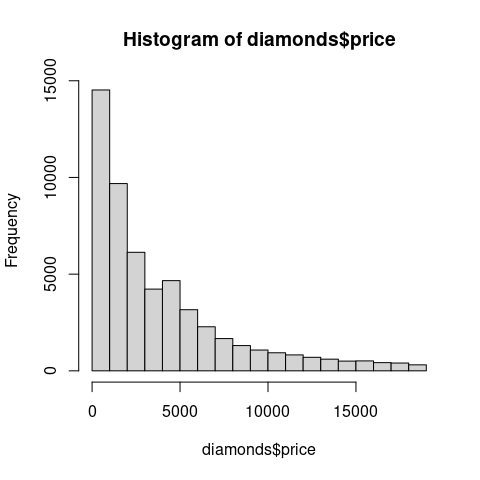# How many unique prices?
length(unique(diamonds$price)) #>  11602 This variable should clearly not be treated as a factor with 11602 levels. To make sure we fit a continuous density for price, we re-class the feature as numeric. # Re-class diamonds$price <- as.numeric(diamonds$price) # Take a random subsample of size 2000 s_idx <- sample(1:nrow(diamonds), 2000) # Train ARF arf <- adversarial_rf(diamonds[s_idx, ]) #> Iteration: 0, Accuracy: 97.04% #> Iteration: 1, Accuracy: 72.16% #> Iteration: 2, Accuracy: 51.72% #> Iteration: 3, Accuracy: 50.49% #> Iteration: 4, Accuracy: 48.06% # Estimate parameters params <- forde(arf, diamonds[s_idx, ]) # Check distributional families params$meta
#>     variable          class    family
#>  1:    carat        numeric truncnorm
#>  2:      cut ordered,factor  multinom
#>  3:    color ordered,factor  multinom
#>  4:  clarity ordered,factor  multinom
#>  5:    depth        numeric truncnorm
#>  6:    table        numeric truncnorm
#>  7:    price        numeric truncnorm
#>  8:        x        numeric truncnorm
#>  9:        y        numeric truncnorm
#> 10:        z        numeric truncnorm

# Forge data, check histogram
synth <- forge(params, n_synth = 1000)
hist(synth$price)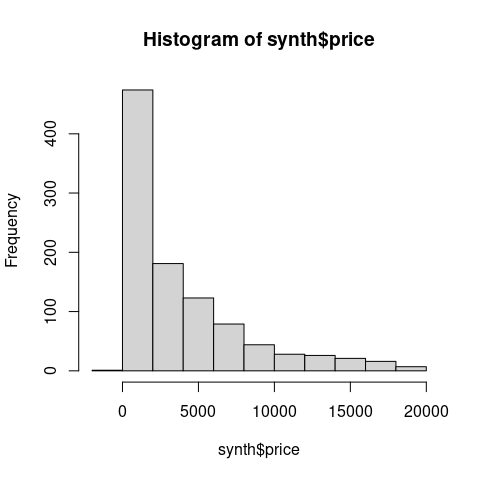Using family = 'truncnorm', the distribution for price will now be modeled with a truncated Gaussian mixture. Though the general outline of the histogram looks about right, we do find some implausible values, e.g. negative prices. This can be overcome by manually setting a hard lower bound. # Set price minimum to empirical lower bound params$cnt[variable == 'price', min := min(diamonds$price)] # Re-forge, check histogram synth <- forge(params, n_synth = 1000) hist(synth$price)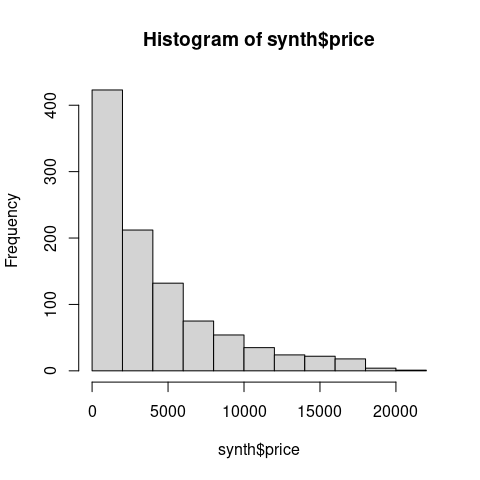Alternatively, we could retrain under log transformation.

# Transform price variable
tmp <- as.data.table(diamonds[s_idx, ])
tmp[, price := log(price)]

# Retrain ARF
#> Iteration: 0, Accuracy: 96.49%
#> Iteration: 1, Accuracy: 73.95%
#> Iteration: 2, Accuracy: 52.15%
#> Iteration: 3, Accuracy: 51.04%
#> Iteration: 4, Accuracy: 50.69%
#> Iteration: 5, Accuracy: 48.4%

# Estimate parameters
params <- forde(arf, tmp)

# Forge, check histogram
synth <- forge(params, n_synth = 1000)
hist(exp(synth\$price))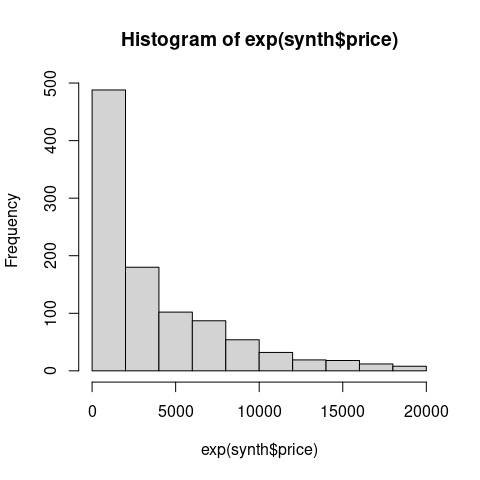This may be unnecessary with sufficiently large sample sizes. For instance, negative prices are exceedingly rare when training on the complete diamonds dataset ($$n = 53940$$). However, if natural constraints are known a priori, they can easily be incorporated into params.